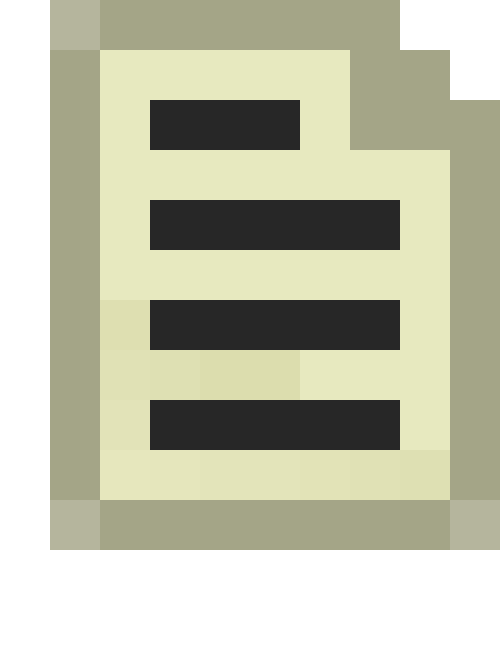# Field of View

Field of view (FOV) is the maximum angle between the peripherals of a person's or camera's vision.

In HL2 the Player Character's Horizontal Field of View is set to 75 degrees. Screen settings aspect ratios are achieved by cropping the top and bottom of the image (ie reducing the vertical FOV).

The NPC FOV angle determines the 'scope' of their Viewcone.

The "fov" console command is flagged as a cheat; to alter the player's FOV via map entities, use env_zoom.

## Optimising Player FOV

• Motion Sickness : Smaller FOVs tend to exaggerate camera movement, whilst larger FOVs tend to minimise its effect on the image. Thus setting a wider FOV can sometimes help to reduce Motion Sickness during gameplay. If you are experiencing such problems in Half-Life 2, try changing the FOV to 90 using the command "`sv_cheats 1;fov 90`" in the console.
• Perspective Distortion : Wider FOVs introduce more Perspective Distortion into a image, whereas small FOVs tend to flatten perspective. The distortion is caused by projecting (spherical) optical geometry onto a flat screen - that's why IMAX Dome screens are curved. Like all optical artifacts, perspective distortion is much more noticeable in still images than in the moving images of FPS gameplay. For making more cinematic screenshots, use a smaller FOV and a larger camera-object distance.
• Theoretically, an optically correct perspective can be obtained by matching the Camera's angle of view to the angle between the Player's eye and the edges of the image on his screen. Obviously this angle varies according to the actual size of the Player's screen and how far away from the screen he is actually sitting. Most players will be sitting close to a fairly small screen and unconsciously move their head position to obtain the most comfortable perspective. However, if using an unusually large screen (eg Overhead Projection) a suitable seat position can be calculated with :
```viewer distance = (screenwidth)/(2*tan(FOV/2))
```

so where the FOV is 75 degrees:

```viewer distance = (screenwidth * 0.652)
```

## FOV calculations

• A useful rule of thumb: an object will appear twice as tall and twice as wide if the camera-to-object distance is halved. This is true for any FOV. This may be helpful when working with LOD distances such as making LOD Models, MIP Mapping textures, and setting Fade Distances.
• To calculate actual screen pixels is usually unnecessary, but quite straight forward:
1. The trick is to bisect the "FOV" and use simple trigonometry to convert the Angle to a ratio of : FrameWidth / Distance = 2 (tan (FOV/2). So for the Player's 75° FOV, the FrameWidth = Distance * 1.535.
2. This represents how much the Frame's dimension increases with distance - which is very useful in itself. But we want to know how an object "shrinks" (relative to the frame) over distance, so our ObjectScale is the inverse of the FrameScale, ie : 1/ (2(tan (FOV/2))). For the Player's 75° Angle of View, the ObjectScale is 1/1.535 == 0.652.
3. An object which is 8 units wide and 512 units away from the Player covers ObjectScale * ObjectWidth / Distance == 0.652 * 8 / 512 = 1% of the horizontal FOV, which is 1% of the horizontal screen resolution.
4. Now, if you half the distance or double the size of the object, it will cover 2% of the width of your screen.
5. If your horizontal screen resolution is 1600 pixels, 1% of 1600 = 16 pixels, 2% = 32 pixels, etc.
• If the ObjectWidth is the same as the Distance, then (for Player's 75°) 0.652 * 1/1 = 65%, which is close enough to remember : 64 unit wide object viewed at 64 units distance covers 64% of the screenwidth.
• For 90° Angle of View the ObjectScale = 0.5.
• If you want it all in one formula (which is much less handy) : ObjectScreenWidth = ScreenResolution * ObjectWidth / (ObjectDistance * (2 tan (FOV/2)))Note: In Source, we work to a percentage of Horizontal FOV because Vertical FOV varies with Aspect Ratio settings. However, the ObjectScale in pixels is the same for both ObjectHeight and ObjectWidth.